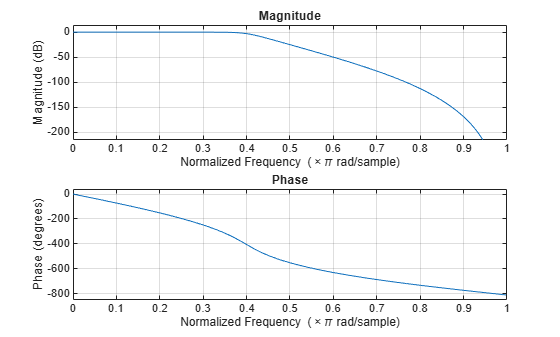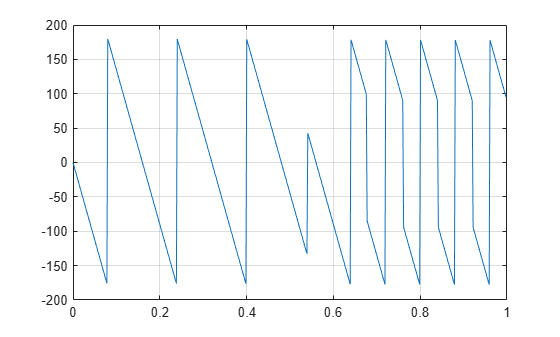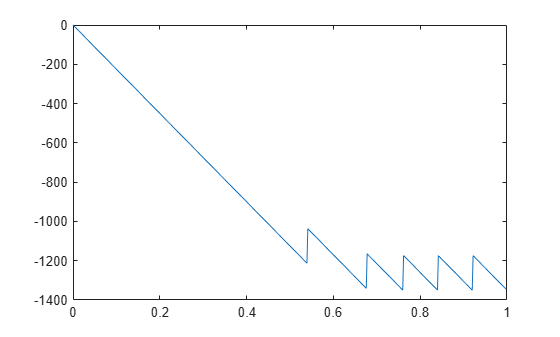Documentation

## Phase Response

MATLAB® functions are available to extract the phase response of a filter. Given a frequency response, the function `abs` returns the magnitude and `angle` returns the phase angle in radians. To view the magnitude and phase of a Butterworth filter using `fvtool`:

```d = designfilt('lowpassiir','FilterOrder',9, ... 'HalfPowerFrequency',400,'SampleRate',2000); fvtool(d,'Analysis','freq')```You can also click the Magnitude and Phase Response button on the toolbar or select Analysis > Magnitude and Phase Response to display the plot.

The `unwrap` function is also useful in frequency analysis. `unwrap` unwraps the phase to make it continuous across 360° phase discontinuities by adding multiples of ±360°, as needed. To see how `unwrap` is useful, design a 25th-order lowpass FIR filter:

`h = fir1(25,0.4);`

Obtain the frequency response with `freqz` and plot the phase in degrees:

```[H,f] = freqz(h,1,512,2); plot(f,angle(H)*180/pi) grid```It is difficult to distinguish the 360° jumps (an artifact of the arctangent function inside `angle`) from the 180° jumps that signify zeros in the frequency response.

`unwrap` eliminates the 360° jumps:

`plot(f,unwrap(angle(H))*180/pi)`Alternatively, you can use `phasez` to see the unwrapped phase:

`phasez(h,1)`